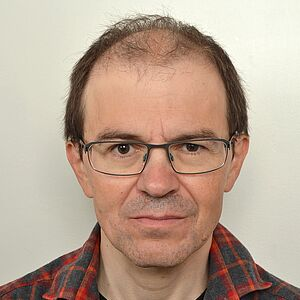## Paramétrage

### Accessibility# BenoitFresse

(+33) 3 20 43 45 71
M2-003
Schools and divisions
Research topics Mathematics Keywords Algebraic topology, Operad theory

## Introduction

I work on the theory of operads and its applications in algebraic topology. An operad is an algebraic structure whose elements represent operations. The usual categories of algebras, such as the associative and commutative algebras, the associative algebras, the Lie algebras and the Poisson algebras, are all governed by operations that generate operads. The notion of an operad has been introduced in topology, in the late 60s, in order to study the structure of iterated loop spaces. Since then, operads have been used fruitfully in other domains and it has become clear that this notion provides both an effective and conceptual device to handle multiple algebraic structures in various contexts.

A core idea of my research is to use modules over operads in order to compare the categories of algebras associated to operads. The most completed version of this research has been published in the book [2009a] of my publication list. The main original result of this book is a general homotopy invariance theorem which asserts that, under standard cofibration assumptions of homotopy theory, the functors associated to modules over operads preserve homotopy equivalences.

The work of Jean-Pierre Serre in the 50s has introduced the idea of using the homology of loop spaces in topology. I have been able to define an algebraic model of iterated loop spaces by using the outcome of my research on modules over operads. By using this result, I have proved in the article [2011c] that the cohomology of an n-fold iterated loop space on a space X can be determined by applying an operadic cohomology theory, defined in purely algebraic terms, to the cochain algebra of the space X. One of the ultimate goals of my research is to use this model to understand the cohomology of certain iterated loop spaces and to determine classes in the homotopy of spaces.

Motivated by this study of algebraic models for the homotopy of iterated loop spaces, I have undertaken a series of research on the homotopy of En operads, the operads that intervein in the definition of these models. These objects, where n=1,2,...,∞ is a dimension parameter, reflect a hierarchy of homotopy commutative structures, from fully homotopy associative but non-commutative (n=1) up to fully homotopy associative and commutative (n=∞). The Goodwillie-Weiss theory of embedding calculus, which proves that the spaces of maps from an Em operad into an En operad determine the homotopy type of the spaces of embeddings between Euclidean spaces, gives another motivation for the study these objects.

In the book [2017a], I have proved that the group of rational homotopy self-equivalences of E2 operads (the group of homotopy classes of rational homotopy automorphisms) is identified with the rational Grothendieck-Teichmüller group (the group introduced by Vladimir Drinfeld in the theory of quantum groups by using insights of the Grothendieck program in Galois theory). The goal of my current research is to go further into the study of the group of homotopy self-equivalences of En operads, for any dimension n≥2 (the case n=1 being trivial), in order to understand internal symmetries of homotopy commutative structures. In this direction, I have proved in a joint work with Victor Turchin (KSU) and Thomas Willwacher (ETHZ) that variants of the Kontsevich graph complexes determine the homotopy type of the spaces of rational homotopy self-equivalences of En operads, for all n≥2, as well as the rational homotopy type of the spaces of maps from an Em operad into an En operad, for n≥m≥1 (see [2017pre]). The next objective of my research is to explore possible generalizations of these results in the positive characteristic framework.